• 2018-07-27 14:24:18
import numpy as np
import math
import matplotlib.pyplot as plt
plt.figure(1)
x=[]
y=[]
a=np.linspace(0,10,100)
def f1(x):
y=1
return y
def f2(x):
y=1/(x*x+1)
return y
def f3(x):
y=math.sin(x)/(math.exp(x)+1)
return y
for i in a:
x.append(i)
y.append(f3(i))
plt.plot(x,y)
plt.show()

更多相关内容
• python绘制函数图像代码，NumPy(Numerical Python) 是 Python 语言的一个扩展程序库，支持大量的维度数组与矩阵运算，此外也针对数组运算提供大量的数学函数库。 NumPy 的前身 Numeric 最早是由 Jim Hugunin 与其它...
• ## Python绘制函数图像

万次阅读 多人点赞 2020-06-03 21:44:09
一元一次函数 一元二次函数 指数函数 正余弦函数 二元一次函数 二元二次函数 导入相关包 from mpl_toolkits.mplot3d import Axes3D import numpy as np from matplotlib import pyplot as plt plt.rcParams...

目录

导入相关包

一元一次函数

一元二次函数

指数函数

正余弦函数

二元一次函数

二元二次函数

## 导入相关包

from mpl_toolkits.mplot3d import Axes3D
import numpy as np
from matplotlib import pyplot as plt
plt.rcParams['font.sans-serif'] = ['SimHei'] #用来正常显示中文标签
plt.rcParams['axes.unicode_minus'] = False #用来正常显示负号

## 一元一次函数

# 一元一次函数图像
x = np.arange(-10, 10, 0.1)
y = 2 * x
plt.xlabel('x')
plt.ylabel('y')
plt.title("一元一次函数")
plt.plot(x, y)
plt.show()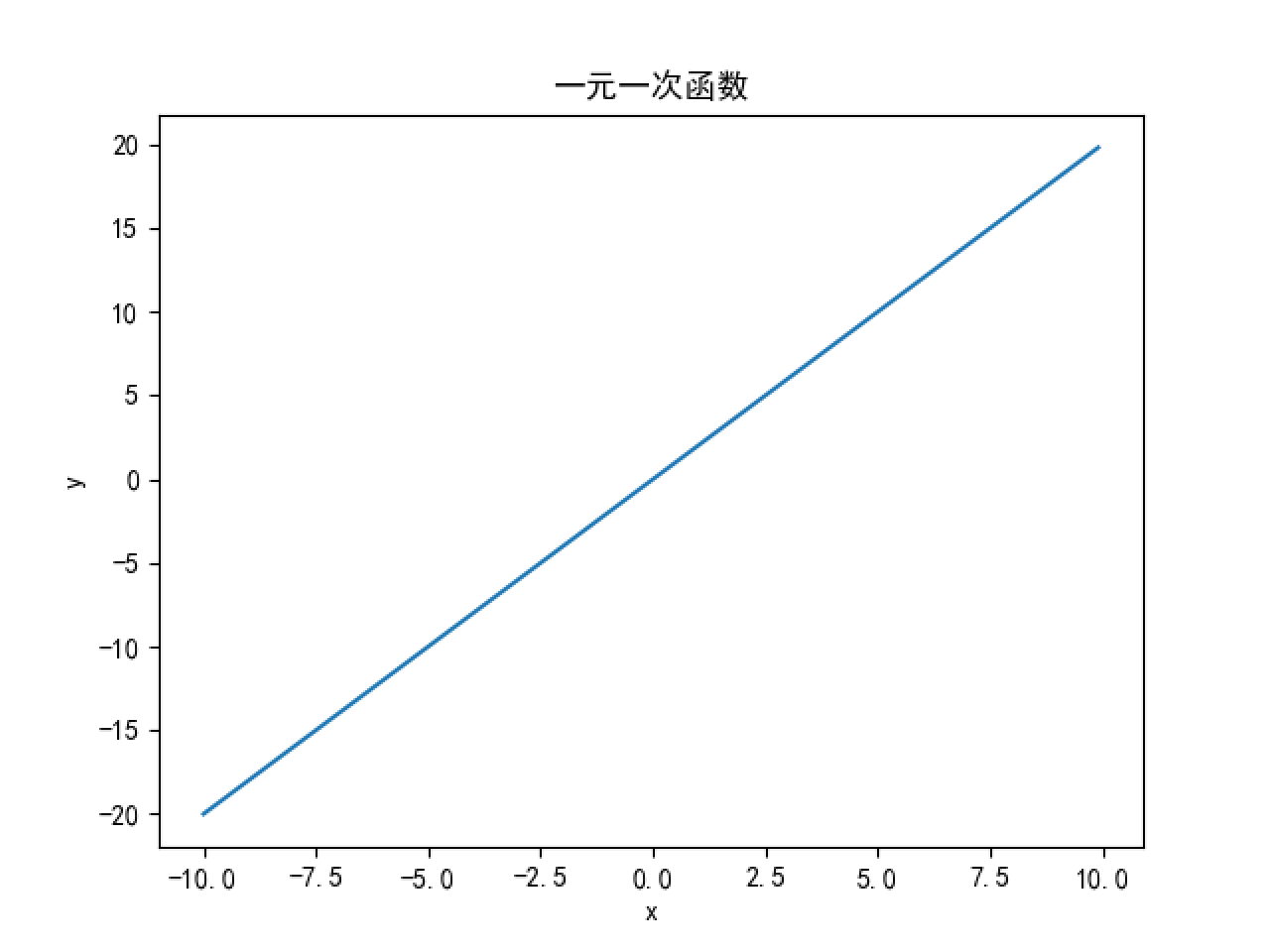## 一元二次函数

# 一元二次函数图像
x = np.arange(-10, 10, 0.1)
y = x * x
plt.xlabel('x')
plt.ylabel('y')
plt.title("一元二次函数")
plt.plot(x, y)
plt.show()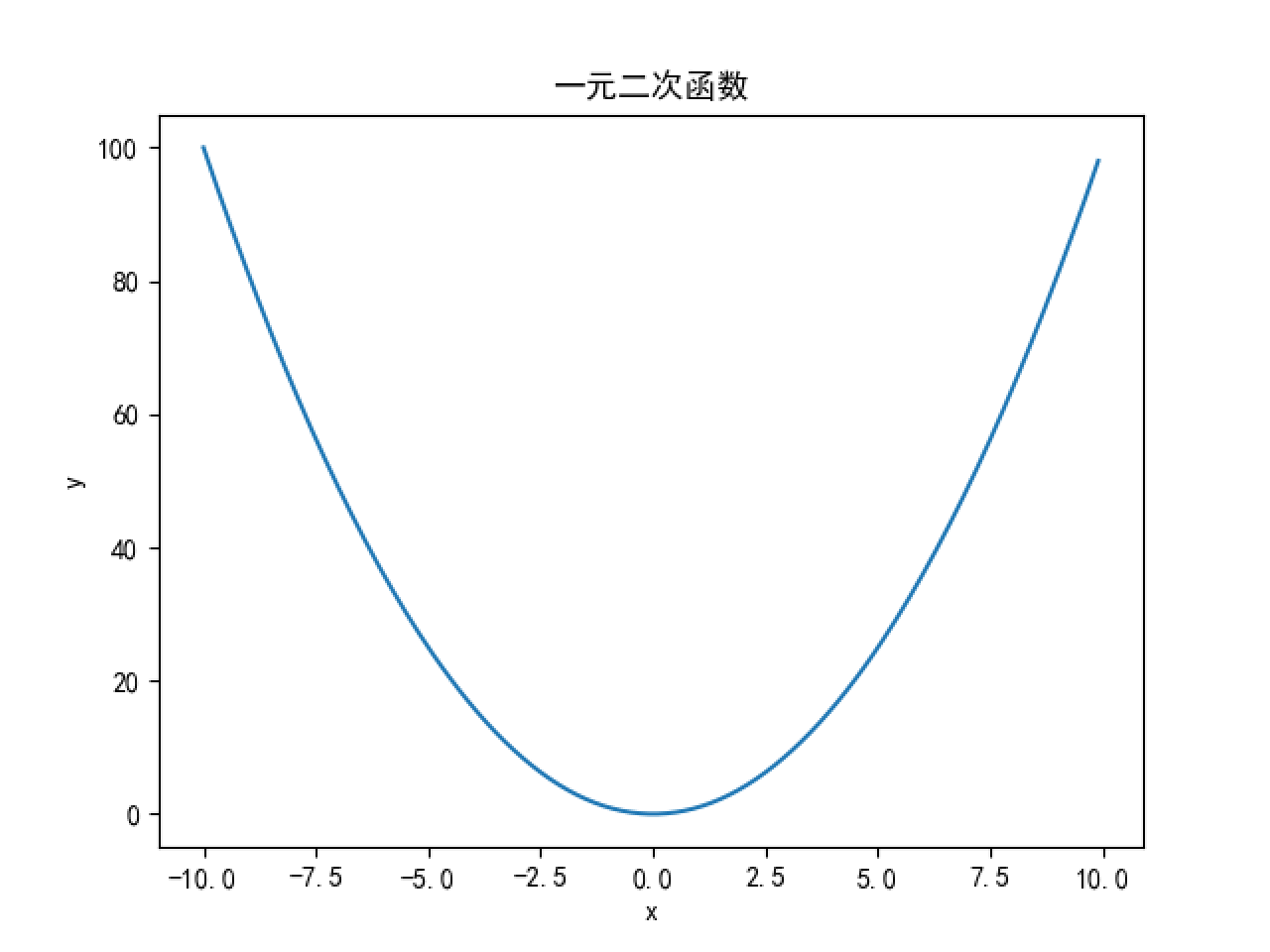## 指数函数

# 指数函数
x = np.arange(-10, 10, 0.1)
y = np.power(2, x)
plt.xlabel('x')
plt.ylabel('y')
plt.title("指数函数")
plt.plot(x, y)
plt.show()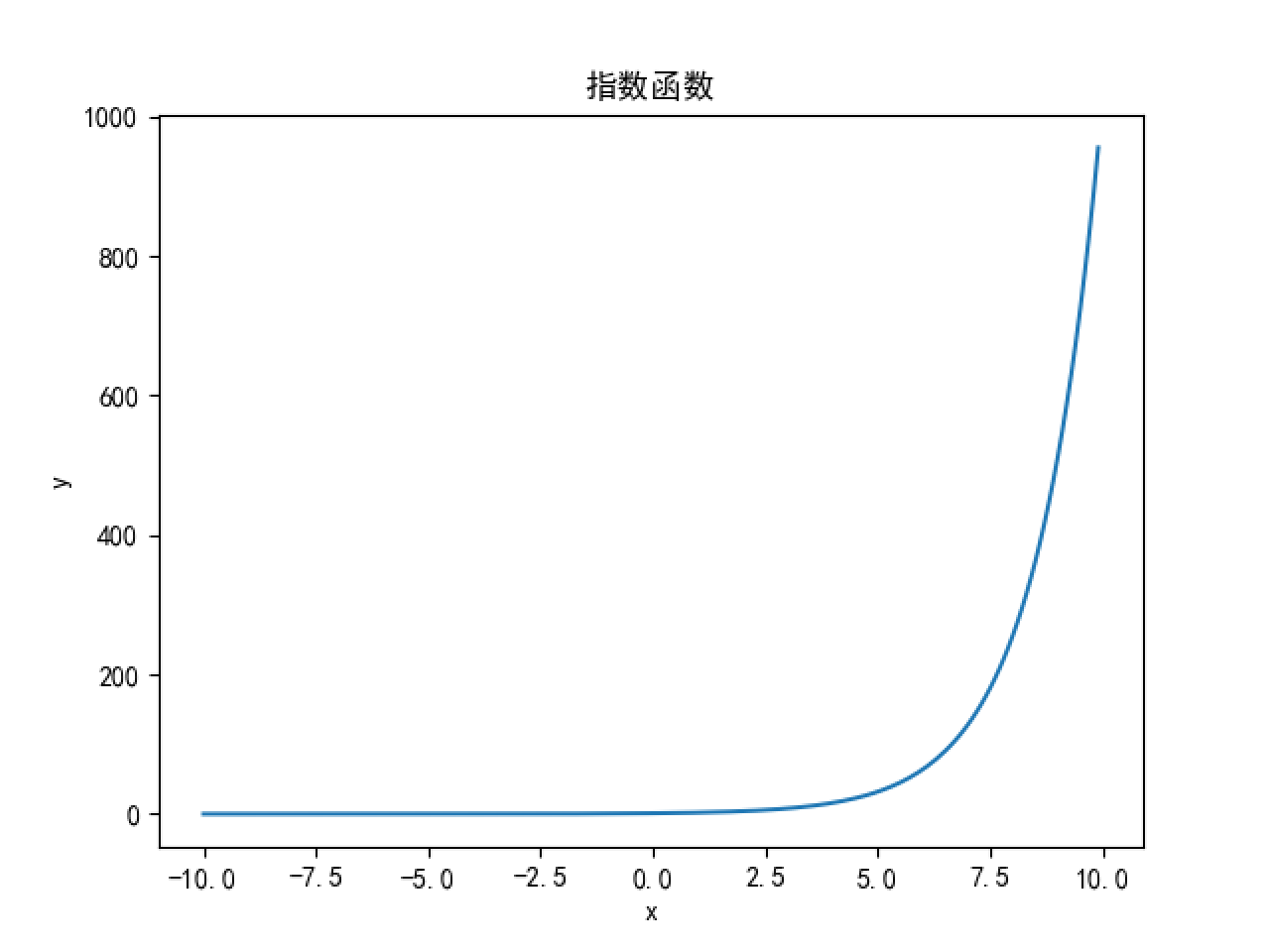## 正余弦函数

# 正弦函数
x = np.arange(-3 * np.pi, 3 * np.pi, 0.1)
y = np.sin(x)
plt.xlabel('x')
plt.ylabel('y')
plt.title("正弦函数")
plt.plot(x, y)
plt.show()

# 余弦函数
x = np.arange(-3 * np.pi, 3 * np.pi, 0.1)
y = np.cos(x)
plt.xlabel('x')
plt.ylabel('y')
plt.title("余弦函数")
plt.plot(x, y)
plt.show()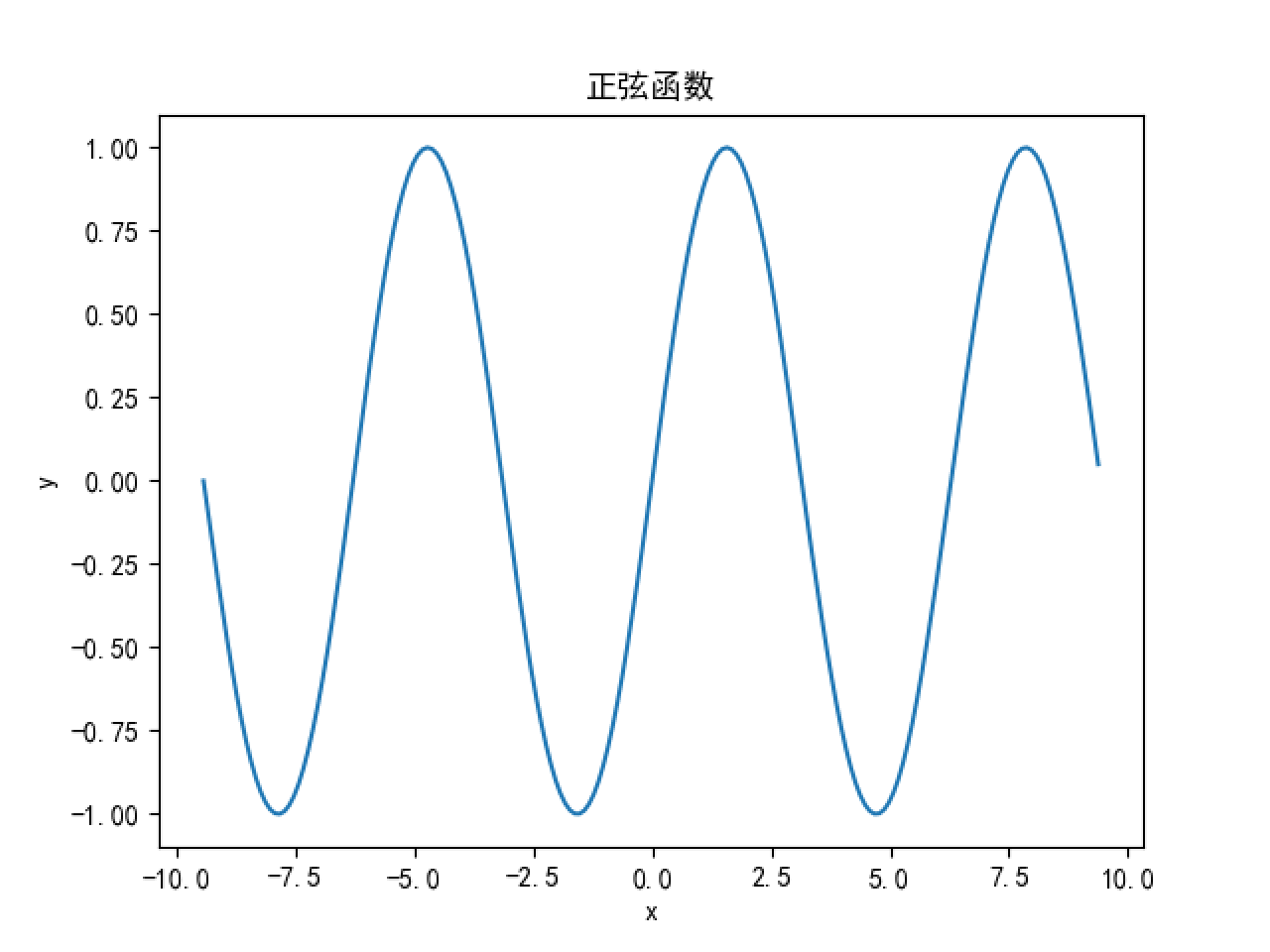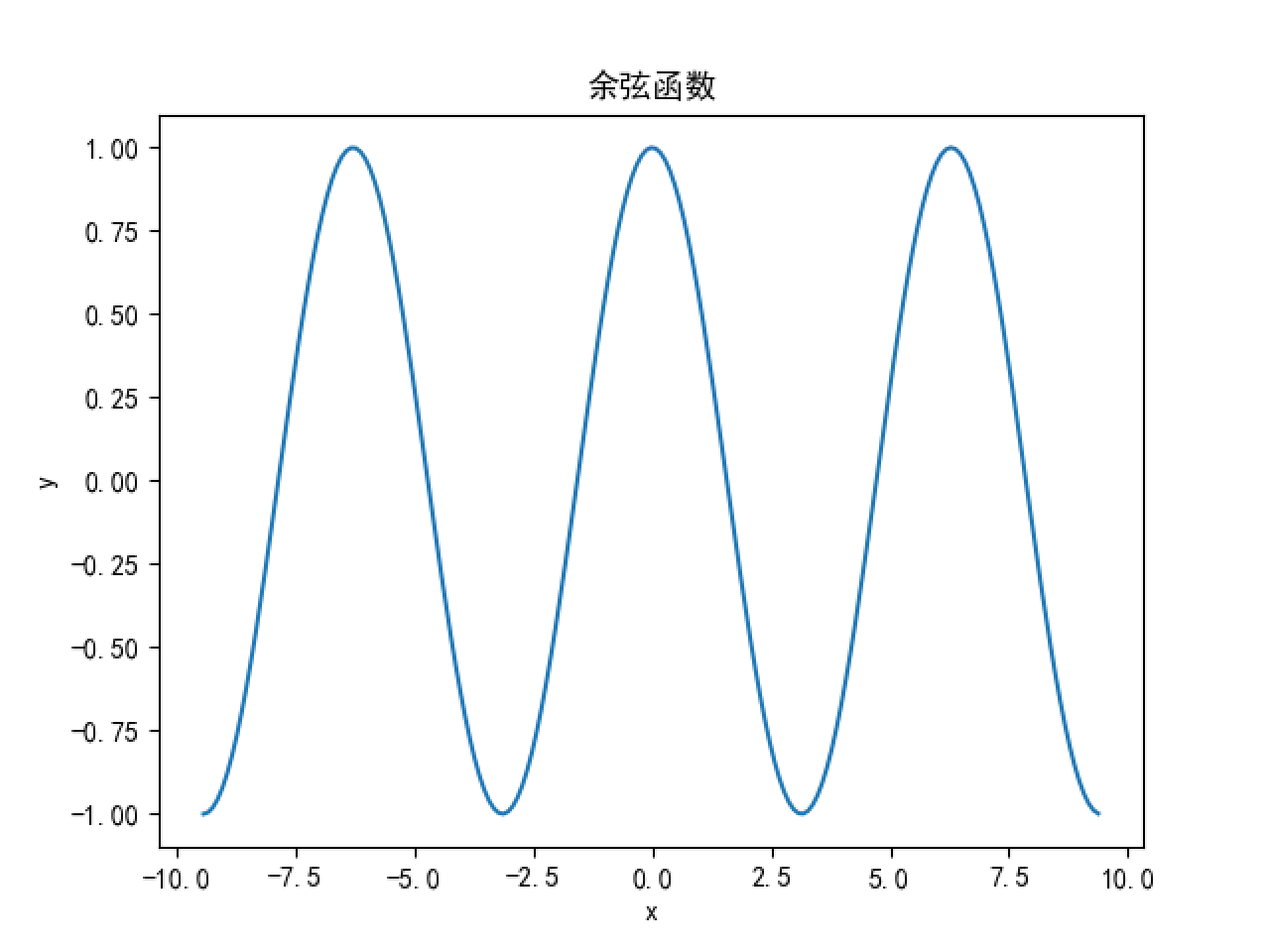## 二元一次函数

#  二元一次函数图像
fig = plt.figure()
ax = Axes3D(fig)
x = np.arange(-100, 100, 1)
y = np.arange(-100, 100, 1)
X, Y = np.meshgrid(x, y)  # 网格的创建，生成二维数组，这个是关键
Z = X + Y
plt.xlabel('x')
plt.ylabel('y')
# 将函数显示为3d,rstride和cstride代表row(行)和column(列)的跨度cmap为色图分类
ax.plot_surface(X, Y, Z, rstride=1, cstride=1, cmap='rainbow')
plt.show()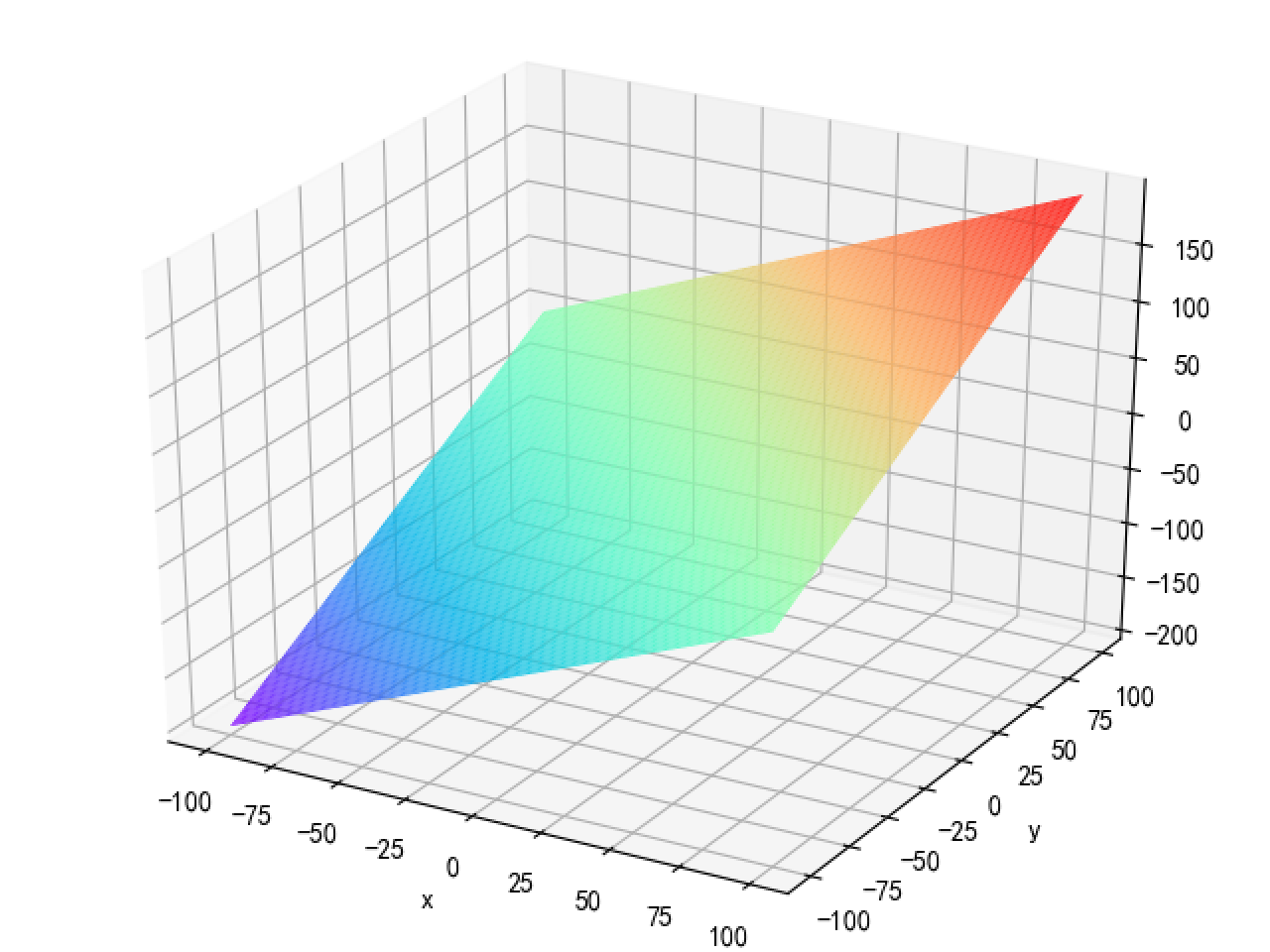## 二元二次函数

#  二元二次函数图像
fig = plt.figure()
ax = Axes3D(fig)
x = np.arange(-100, 100, 1)
y = np.arange(-100, 100, 1)
X, Y = np.meshgrid(x, y)  # 网格的创建，生成二维数组，这个是关键
Z = X*X + Y*Y
plt.xlabel('x')
plt.ylabel('y')
# 将函数显示为3d,rstride和cstride代表row(行)和column(列)的跨度cmap为色图分类
ax.plot_surface(X, Y, Z, rstride=1, cstride=1, cmap='rainbow')
plt.show()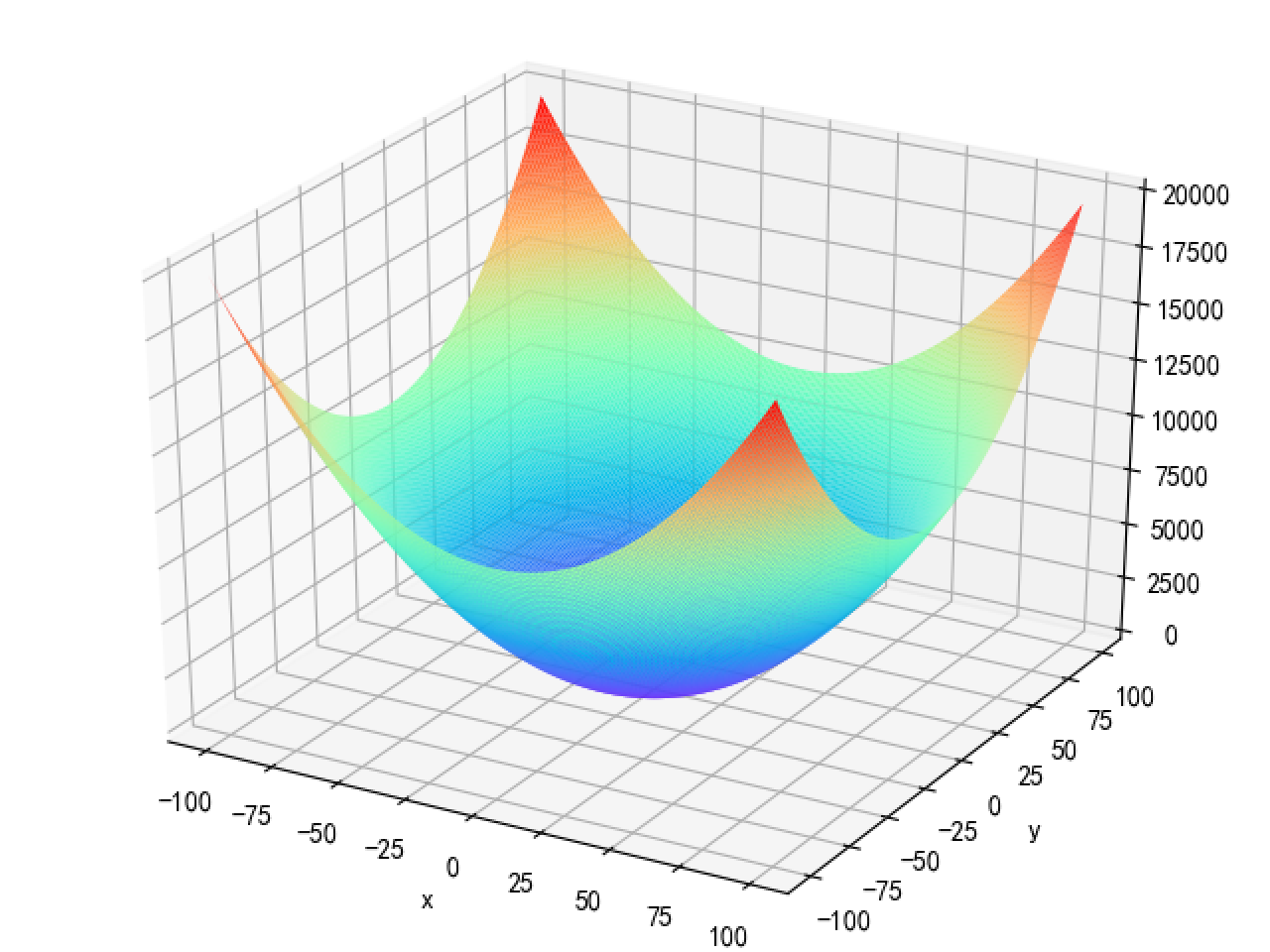展开全文• 今天小编就为大家分享一篇使用python绘制二元函数图像的实例，具有很好的参考价值，希望对大家有所帮助。一起跟随小编过来看看吧
• python实现绘制二次函数图像 python实现绘制三维函数图像 python实现绘制二次函数图像 import matplotlib.pyplot as plt import numpy as np import math x = np.linspace(-10, 10, 10) #分别代表最小，最大，...

目录

python实现绘制二次函数图像

python实现绘制三维函数图像

# python实现绘制二次函数图像

import matplotlib.pyplot as plt
import numpy as np
import math
x = np.linspace(-10, 10, 10)   #分别代表最小，最大，数量， 生成一个等差数列
y = x ** 2 + 1
plt.plot(x, y)
plt.show
展开全文开发语言 后端
• 在学习数学时，经常希望绘制2/3D的函数图像/图像帮助理解 以下是实现方法： 2D图像: from matplotlib import pyplot as plt import numpy as np from mpl_toolkits.mplot3d import axes3d low=lambda x:...

在学习数学时，经常希望绘制2/3D的函数图像/图像帮助理解
以下是实现方法：

## 2D图像:

from matplotlib import pyplot as plt
import numpy as np
from mpl_toolkits.mplot3d import axes3d

low=lambda x:10000 if x>10000 else -10000 if x<-10000 else x

f=lambda x:x**5#设计一个函数

start=-10 #输入需要绘制的起始值（从左到右）
stop=10 #输入需要绘制的终点值
step=0.01#输入步长

num=(stop-start)/step #计算点的个数
x = np.linspace(start,stop,num)
y = f(x)

for i in range(len(y)):#再应用一个low函数以防止函数值过大导致显示错误（可选）#若函数无法直接应用到np数组上可以使用for来逐个应用
y[i]=low(y[i])
z=y

fig=plt.figure(figsize=(6,6))#建立一个对象并设置窗体的大小，使其为正方形，好看 #注意 可以建立多个对象，但plt指令只会对最后一个指定的对象进行操作（查看过源码了）

plt.plot(x, y,label='First Curve')#在当前的对象上进行操作

plt.grid(True)#显示网格

plt.axis("equal")#设置了x、y刻度长度一致#需要放在x、ylim指令前
plt.xlim((-10, 10))#显示的x的范围（不设置则由程序自动设置）
plt.ylim((-10, 10))#显示的y的范围

plt.plot([2*min(x),2*max(x)], [0,0],label='x-axis')#用定义域最长距离的两倍作出x轴
plt.plot([0,0],[2*min(y),2*max(y)],label='y-axis')#用值域最长距离的两倍作出y轴
plt.legend()#显示旁注#注意：不会显示后来再定义的旁注
plt.show(fig)#没有输入值默认展示所有对象 #注意：plt.show()之后再次使用plt.show()指令将不会展示任何对象，若想再次展示对象，可以对对象使用fig.show()


效果如图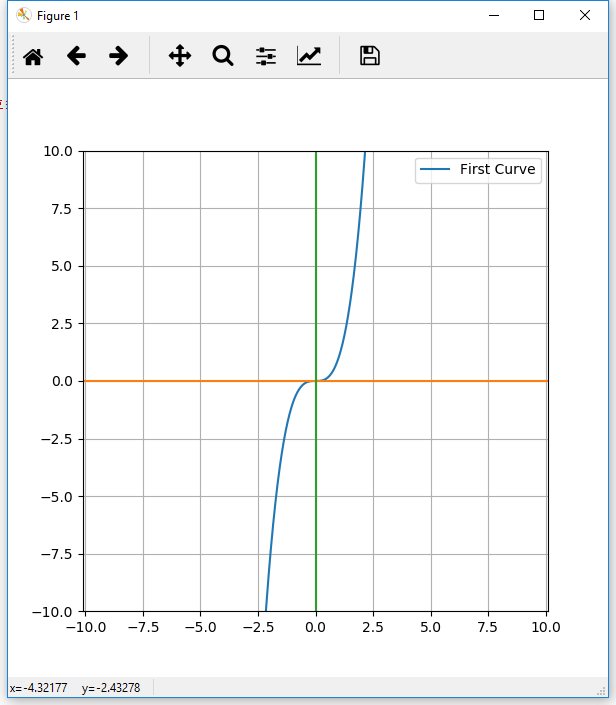## 3D图像

一、3D曲线

import numpy as np  #载入numpy
import matplotlib.pyplot as plt  #载入matplotlib.pyplot
from mpl_toolkits.mplot3d import Axes3D  #载入Axes3D用以实现3D作图

fig=plt.figure(figsize=(6,6)) #创建一个对象（正方形窗体）#注意由于3D作图是设置'equal'没什么效果，且3D作图的缩放是等比例缩放所以只需要设定窗体为正方形并且不去变动窗体形状就可以保证x、y、z刻度长度一致
ax=Axes3D(fig) #设置这个对象为3D作图的

t=np.linspace(-1,1,100)#设置一个参数t

#设计一个单变量的参数方程
x=t
y=t**3
z=t

ax.plot(x,y,z,label='First Curve') #用ax进行3D作画

m=max(max(x),max(y),max(z))#求出x,y,z三个集合中的最大元素

#用xyz中的最大元素建立直角坐标系，由于此时xyz三个方向上的最大最小值大小都相同，→此时xyz三轴的刻度长度是相等的（一举两得）
ax.plot([-m,m],[0,0],[0,0],label='x-axis')
ax.plot([0,0],[-m,m],[0,0],label='y-axis')
ax.plot([0,0],[0,0],[-m,m],label='z-axis')

ax.legend()
ax.set_xlabel('x axis')#标注x、y、z轴帮助观察
ax.set_ylabel('y axis')
ax.set_zlabel('z axis')
plt.show()


值得注意的是：在上述两例中均使用的是plot函数来进行线性制图，这会将每个点按顺序连接起来，而我们也可以直接使用scatter函数（plt.scatter或ax.scatter）来进行散点制图。散点制图额外的优点是不会在函数数值极大时导致极远处的两个点被连成一条线影响美观影响判断

二、3D曲面

from matplotlib import pyplot as plt
import numpy as np
from mpl_toolkits.mplot3d import Axes3D

fig = plt.figure()
ax = Axes3D(fig)
x = np.arange(-2, 2, 0.1) #创建等差数组 与np.linspace类似但不相同（arange使用的是步长不是步数）
y = np.arange(-2, 2, 0.1)
x, y = np.meshgrid(x, y)
#meshgrid生成两个新的矩阵，其中前一个矩阵中的每一个元素都在后一个矩阵中的相同位置有一个对应，且这个对应满足x中的每一个元素都与y中的每一个元素对应一次，这种对应的总数是x与y的元素数量之积，可以转化为一种组合。
#在建立曲面的时候，我们需要两个变量（在参数方程中意为我们需要两个参数）。当这两个变量是有限个的时候我们需要分别考虑他们的每一种组合。meshgrid便提供了实现这件事的简便方法。

z = x**2 + y**2-5 #这是一个经过平移的抛物面的方程，我们可以直接对x和y进行组合并求z来得到一个近似的抛物面图像（我们也可以把这个方程改写成参数方程并用参数的组合来得到一个近似的抛物面图像） #对矩阵**2并相加可以使对应元素各自平方并求和

ax.plot_wireframe(x, y, z,label='First Surface')  #plot_wireframe可以创建网格式的平面（plot_surface可以创建平面但是程序运行较慢,适合截图不适合拖动）

m=max(abs(x).max(),abs(y).max(),abs(z).max())#求出x,y,z三个集合中的最大元素

#用xyz中的最大元素建立直角坐标系，由于此时xyz三个方向上的最大最小值大小都相同，→此时xyz三轴的刻度长度是相等的（一举两得）
ax.plot([-m,m],[0,0],[0,0],label='x-axis')
ax.plot([0,0],[-m,m],[0,0],label='y-axis')
ax.plot([0,0],[0,0],[-m,m],label='z-axis')

ax.set_xlabel('x axis')#标注x、y、z轴帮助观察
ax.set_ylabel('y axis')
ax.set_zlabel('z axis')
plt.show()



3D曲面效果如图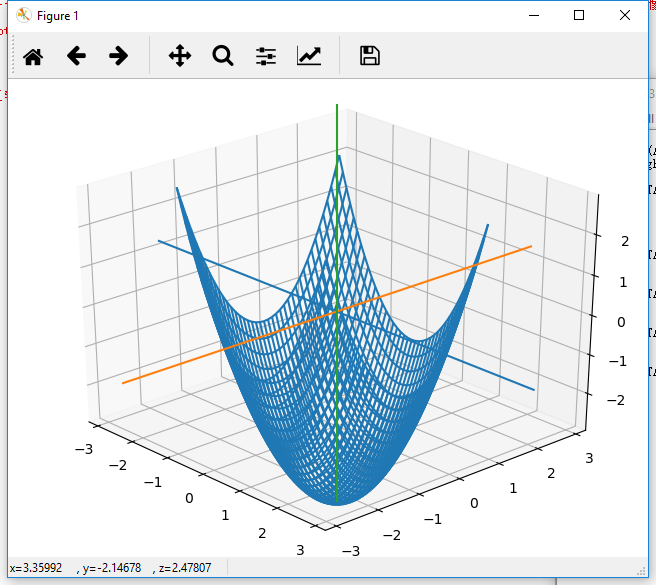总结：
1.matplotlib是一个复杂的功能强大多样的库，我们可以从中各取所需，不一定要学习透彻。
2.由于函数与图像是多样的，所以在使用matplotlib辅助学习时要灵活调整其中的参数，不一定要与本文中的例子完全一样。

更多matplotlib的使用方法见：http://blog.csdn.net/wizardforcel/article/details/54407212

展开全文• ## 用Python绘制数学函数图像

万次阅读 多人点赞 2021-05-26 16:13:23
博客文章: ...答案是有很多的，有很多不错的软件都能画出函数图像，但是，我想到了Python的数据可视化。Python在近些年非常火热，在数据分析以及深度学习等方面得到广泛地运用，其丰富的库使其功能愈.numpy
• 需要的库：matplotlib用法：importmatplotlib.pyplot as pltplt.plot(x, y) #x， y是两个列表plt.show()例子：importmatplotlib.pyplot as pltx= [1, 2, 3]y= [2, 3, 4]...实际上，x， y的点越多，图像越精确，我们...
• 最近因为复习高数在网上找三角函数图像，发现大多数都模糊不清，实在是头痛，所以自己学以致用，用Python画出了三角函数图像。希望我的博客可以帮到大家，也祝考研党们早日上岸，考上理想的学校！ 想了解代码的详细...
• 主要介绍了Python绘制正余弦函数图像的方法，小编觉得挺不错的，现在分享给大家，也给大家做个参考。一起跟随小编过来看看吧
• 1.绘制2D简单函数 import numpy as np import matplotlib.pyplot as plt x=np.linspace(0,4*np.pi,100) #从0到4π均匀取100个值组成数组 wave=np.cos(x) #对x数组的每个数进行cos运算，算出来的值组成数组 plt.plot...图像处理
• ## 用python画函数图像

千次阅读 2020-12-18 22:40:41
画sigmoid激活函数： import numpy as np import matplotlib.pylab as plt def sigmoid(x): return 1/(1+np.exp(-x)) # sigmoid函数 x = np.arange(-6.0,6.0,0.1) # 限定x的范围，给什么区间画出来的就是在哪个...开发语言 后端
• 今天小编就为大家分享一篇python/Matplotlib绘制复变函数图像教程，具有很好的参考价值，希望对大家有所帮助。一起跟随小编过来看看吧
• 目的: 发出 y = x ** 3 / (x * 3 + 1) 的图像 1. 导入matplotlib模块还有numpy模块: import numpy as np import matplotlib.pyplot as plt 2. di'y
• ## python绘制函数曲线

万次阅读 多人点赞 2019-09-15 23:21:44
相对来说，python完全免费，只需要安装一个解释器，并且有很多科学计算库可以调用，所以后来就一直使用python来画曲线，记录下最近画的几条曲线。 环境：mac、pycharm、anaconda 1.sigmoid曲线 sigmoid曲线公式如下...曲线 jpeg
• 很多时候我们数据处理的时候要画坐标图，下面我用第三方库matplotlib以及scipy绘制光滑的曲线图 需要安装的库有 matplotlib,scipy, numpy import matplotlib.pyplot as plt import numpy as np from mpl_toolkits....
• 简介本文主要介绍如何通过pyplot来绘制函数图。主要绘制函数如下： - 一元一次函数 - 一元二次函数 - 指数函数 - 自然对数函数 - 正弦函数一元一次函数代码import matplotlib.pyplot as pltimport numpy as npx = np...
• 使用Python绘制二元函数f(x) = (x1)2 + (x2)2的图像。 import numpy as np import matplotlib.pyplot as plt from mpl_toolkits.mplot3d import Axes3D fig = plt.figure() ax = Axes3D(fig) x1 = np.arange(-...机器学习 开发语言
• 利用Python的Matplotlib库可以实现多元函数的可视化，以下以一段代码说明: 第三方库需求 Matplotlib（数据可视化） numpy（数学计算库） 代码 from matplotlib import pyplot as plt import numpy as np from mpl_...
• 下面我们尝试用Python的2D绘图库matplotlib来绘制函数图像。下面我们来实现一个简单的函数：首先，调用matplotlib库和numpy库import matplotlib.pyplot as pyplotimport numpy然后，确定绘制图像的定义域范围（例如...
•机器学习 数据挖掘
• 今天打算通过绘制正弦和余弦函数，从默认的设置开始，一步一步地调整改进，让它变得好看，变成我们初高中学习过的图象那样。通过这个过程来学习如何进行对图表的一些元素的进行调整。 简单绘图 matplotlib有一套允许...
•深度学习 人工智能 神经网络
• 利用matplotlib和numpy库绘制三角函数图像，包括正弦函数、余弦函数、正切函数、余切函数图像 代码如下： import numpy as np import matplotlib as mp import matplotlib.pyplot as plt from matplotlib.font_...matplotlib numpy...